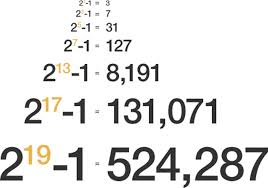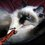# Really neat proof of the infinitude of primes! Why didn't I think of it?Here is a really neat proof of the infinitude of primes! It's so simple that it makes me say: "Why didn't I think of it?". In fact, it was made just in 2005, by Filip Saidak, and I was really surprised to know that. So here is the proof:

Let $n > 1$ be a positive integer. Since $n$ and $n+1$ are consecutive integers, they must be coprime, and hence the number $N_2 = n(n + 1)$ must have at least two different prime factors. Similarly, since the integers $n(n+1)$ and $n(n+1)+1$ are consecutive, and therefore coprime, the number $N_3 = n(n + 1)[n(n + 1) + 1]$ must have at least 3 different prime factors. This can be continued indefinitely.Note by حكيم الفيلسوف الضائع
6 years, 11 months ago

This discussion board is a place to discuss our Daily Challenges and the math and science related to those challenges. Explanations are more than just a solution — they should explain the steps and thinking strategies that you used to obtain the solution. Comments should further the discussion of math and science.

When posting on Brilliant:

• Use the emojis to react to an explanation, whether you're congratulating a job well done , or just really confused .
• Ask specific questions about the challenge or the steps in somebody's explanation. Well-posed questions can add a lot to the discussion, but posting "I don't understand!" doesn't help anyone.
• Try to contribute something new to the discussion, whether it is an extension, generalization or other idea related to the challenge.

MarkdownAppears as
*italics* or _italics_ italics
**bold** or __bold__ bold
- bulleted- list
• bulleted
• list
1. numbered2. list
1. numbered
2. list
Note: you must add a full line of space before and after lists for them to show up correctly
paragraph 1paragraph 2

paragraph 1

paragraph 2

[example link](https://brilliant.org)example link
> This is a quote
This is a quote
    # I indented these lines
# 4 spaces, and now they show
# up as a code block.

print "hello world"
# I indented these lines
# 4 spaces, and now they show
# up as a code block.

print "hello world"
MathAppears as
Remember to wrap math in $$ ... $$ or $ ... $ to ensure proper formatting.
2 \times 3 $2 \times 3$
2^{34} $2^{34}$
a_{i-1} $a_{i-1}$
\frac{2}{3} $\frac{2}{3}$
\sqrt{2} $\sqrt{2}$
\sum_{i=1}^3 $\sum_{i=1}^3$
\sin \theta $\sin \theta$
\boxed{123} $\boxed{123}$

Sort by:

clap clap clap

- 8 months, 4 weeks ago

the interesting thing of primes is that we could come with even simpler proofs of primes and extricating proofs too of primes simpler than that proof of infinite primes infinitude of primes by euclid and still after 2000 years gone by after euclid

- 2 years, 10 months ago

Still on schedule for launch on November 30

- 1 year, 5 months ago

If $\frac{n-1}{\phi(n)}$ is an integer then prove that no prime p gives integer value when $\frac{n}{p^2}$.

- 2 years, 10 months ago

That's really nice!!!

- 3 years ago

I wish to know the proof of this geometric problem. Given a scalene triangle on the sides of which are drawn equilateral triangles having the sides of the given triangle as a side. Prove that the triangle formed by connecting the center of gravity of the three equilateral triangle is equilateral.

- 3 years, 3 months ago

I was unable to sow the proof of this geometric problem. I wish someone can help me.

- 3 years, 3 months ago

2^prime-1= prime..That means there r infinite prime no.s

- 5 years ago

$2^p-1$ is not always prime for $p$ being prime. Eg : $2^{11}-1=2047=23 \times 89$. So, your statement does not actually prove infinite number of primes.

Euclid proves the same by induction over 2000 years ago

- 5 years, 4 months ago

Isn't it proof by contradiction ?

- 3 years, 8 months ago

It seems that the proof shown is for infinitude of composite numbers. I fail to see how it shows that there are infinite number of primes,using this proof??

- 5 years, 11 months ago

if continued indefinitely, the proof is saying that there is a never ending list of composite numbers with a never ending list of unique prime factors. It is different than a proof by contradiction of a largest prime, and is more like a proof of an infinite number of prime factors

- 5 years, 8 months ago

dang

- 6 years, 2 months ago

A different proof also exists for the almost same result and with the same concept , apparently.

The proof goes as -

Let us suppose that there are some finite primes $n$ , suppose. Let the primes be $a_{1} , a_{2} , \ldots , a_{n}$ such that $a_{1} = 2 , a_{2} = 3$ and so on. We can clearly conclude that any number is product of some powers of different primes , for example , $12 = 2^{2} \times 3$. This would mean that every number after $a_{n}$ is a multiple of different powers of different primes or any number $y = a_{1}^{x_{1}} \times a_{2}^{x_{2}} \ldots$ where $x_{1}$ or $x_{z}$ could be any whole number. But here we try to bring contradiction , suppose there exists a number $b$ as it would surely exist as numbers are infinite , where $b=a_{1} \times a_{2} \times a_{3} \ldots a_{n} + 1$. Here , now $a_{1}, \ldots , a_{n}$ would not be divide $b$ but leave a remainder of 1 . This contradicts our hypothesis and hence, we are forced to conclude that primes are infinite

Why didn't I think of it !

- 6 years, 6 months ago

really

- 6 years, 6 months ago

:)

- 3 years, 3 months ago

Amazing!

- 6 years, 6 months ago

Thas' a cool rearrangement o' what we learn in school....

- 6 years, 9 months ago

That's really nice!!!

Darn I have to come up with something like that. :P

- 6 years, 11 months ago